×
Get Full Access to MSU - CEM 141 - Study Guide - Midterm
Get Full Access to MSU - CEM 141 - Study Guide - Midterm

×

MSU / Chemistry / CEM 141 / cem 141 msu exam 2

# cem 141 msu exam 2 Description

##### Description: Covers key concepts from all 14 lectures for EXAM 2, as well as important concepts from the mock exam.
25 Pages 9 Views 24 Unlocks
Reviews

CEM 141 EXAM 2 STUDY GUIDE CHAPTER 2

## who postulated that light must come in packets of photons?Light has wave­particle duality – meaning that it can behave as a wave OR a particle

Light as a Wave

∙ Electromagnetic radiation can be described as a wave composed of oscillating  magnetic and electric fields in perpendicular planes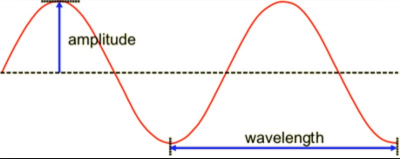∙ Wavelength: the distance between any 2 identical points on neighboring waves ∙ Amplitude: the distance from the average value to any crest or trough

∙ Frequency: the number of oscillations made by a wave in each second

## what is light from one particular element contains only a few of the wavelengths?∙ c =   *  λ υ c is the speed of light in a vacuum (3.0 * 108 m/s),   is the wavelength  λ in meters,   is the frequency in Hertz υ

∙ Greater amplitude indicates greater intensity

∙ Greater frequency indicates greater energy

∙ Wavelength and frequency have an inverse relationship

∙ Electromagnetic spectrum (logarithmic scale):∙ In order to detect an object, the wavelength used must be smaller than the object itself

## what is valence electrons?∙ Evidence that light is a wave: when light shines through a single slit, it diffracts and  forms a band of intensity, but when it shines through two slits, it forms infinite bands  of intensity

∙ Reasoning that light is a wave: since it forms infinite bands of intensity after passing  through two slits, that indicates that light undergoes constructive and destructive  interference to form the light and dark bands ­­­ only waves can have interference (not particles)

∙ Constructive interference: amplitude doubles If you want to learn more check out math 1730

∙ Destructive interference: crests and troughs are cancelled out

Light as a Particle

∙ Wave nature of light does not explain the photoelectric effect

∙ Photoelectric effect: many metals emit electrons when EM radiation shines on the  surface ­­­ the light transfers energy at the surface of the metal to the electrons as  kinetic energy, allowing the electrons to be ejected from the metal (solar panels are an application of this)

∙ Evidence that light is a particle: for the photoelectric effect, there is a threshold  frequency below which no electrons will be emitted (no matter the light intensity) ∙ Reasoning that light is a particle: if light were a wave, increasing the intensity should  cause more electrons to be ejected, without any threshold in frequency ∙ Einstein postulated that light must come in packets of photons to explain this  phenomenon

∙ Planck­Einstein relation: E=h  ­­ where h is Planck’s constant (6.626*10 υ­34 m2*kg*s 1)

∙ Planck postulated that light must quantized energy that is related to frequency ∙ Putting together both equations so far:

Absorption and Emission

∙ Atomic emission spectra: light from one particular element contains only a few of  the wavelengths

∙ Atomic emission spectra are generated by putting the element in a gas discharge  tube, and projecting the emissions through a slit and a prism, and then projecting  the emissions onto a screen

∙ Atomic absorption spectra: include all wavelengths of the visible spectrum that  are not included in the wavelengths of the emission spectra (complementary to  emission spectra)

∙ Generated by shining a hollow cathode lamp through a lens at an atomized sample that is projected to a monochromatorDon't forget about the age old question of bject permanence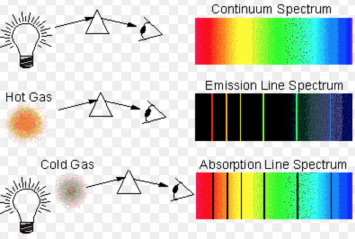∙ Main difference: emission spectra are generated using hot gas, absorption spectra  are generated using cold gas

∙ Each element has characteristic wavelengths that it can absorb or emit ∙ Niels Bohr proposed the idea that electrons had orbits with definite energies and  definite distances from the nucleus (definite distances idea is false) ­­­ he therefore stated that the energies of electrons were quantized

∙ When photons are absorbed, electrons are excited and move up in energy level;  when photons are emitted, electrons are desexcited and electrons move down in  energy level

∙ Energy of the photons corresponds to the difference in the energy levels of the  electrons

∙ To visualize electron energy levels, it is better to use energy diagrams ∙ Electrons transition between energy levels by absorbing or emitting photons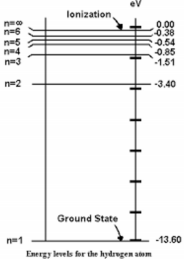We also discuss several other topics like what is the limiting reagent in malachite synthesis

∙ Larger energy jumps in absorption/emission spectra indicate higher  frequency/shorter wavelength light

∙ Energy levels for electrons have negative values because they represent potential  energy from attractive interactions (the PE curve for attractive interactions lies  below the x­axis)

∙ Bohr’s model does not take into account the wave­particle duality of electrons ∙ de Broglie postulated that all matter has wavelengths that can be calculated (wave  properties)   = h/mv λ

∙ For macroscopic objects, the wavelength is much smaller than the size of the  object – so there are no effects on the objects properties due to the wavelengths ∙ For electrons, the wavelength is roughly the size of atoms – affects properties ∙ https://www.youtube.com/watch?v=DfPeprQ7oGc ∙ If you observe a quantum event, you will disturb it significantly Don't forget about the age old question of nau google docs

∙ Heisenberg uncertainty principle: cannot observe both the position and  momentum of a small particle (electrons)

∙ Therefore, Bohr’s model does not hold because the energy and position of the  electrons is impossible to know We also discuss several other topics like emc mizzou

∙ Erwin Schrödinger: created mathematical descriptions of the energies and  probabilities of electrons being in a certain position

∙ Mathematical description of an electron: wave function ( ) Ψ

∙ Probability of finding an electron: (Ψ2)

Orbitals and Electron Configurations

∙ Atomic orbitals: regions of high probability for finding electrons

∙ Each orbital can be described as a set of quantum numbers that are derived from  quantum mechanical calculations

∙ n (principle quantum number) ­­­ determines energy of the electron and describes  its shell

∙ l (angular momentum quantum number) ­­­ determines the type of orbital (s, p, d)  and the subshell

∙ ml (magnetic quantum number) ­­­ determines a particular orbital in a subshell ∙ ms is the spin quantum number ­­­ determines the spin of the electron in each  orbital (+1/2 or ­1/2)

∙ A key consequence of quantum mechanics is that you can only have two electrons in each orbital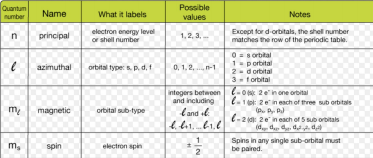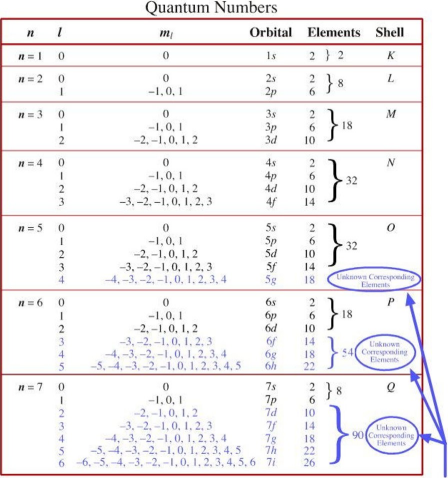∙ S orbitals have l=0, are spherical, and can hold two electrons; 1s is lowest in  energy, 2s is bigger but has the same shape

∙ P orbitals have l=1, have two regions of electron density (lobes), with a planar  node in between

∙ Each type of p orbital lies along a different axis in space

∙ D orbitals have l=2; they have 4 lobes, and are most common among transition  metals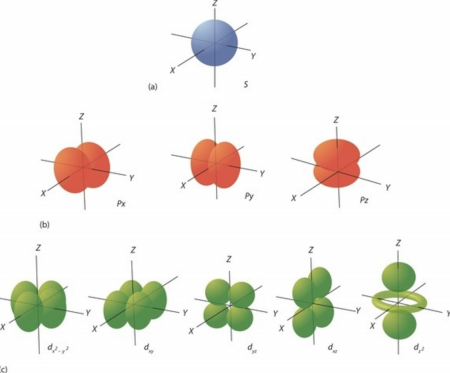∙ Each orbital can contain orbital 2 electrons

∙ Orbitals have different energies∙ Aufbau Principle: electrons occupy lower energy level orbitals first ∙ Elements on the periodic table can be referred to by the location of electrons in  their outermost orbitals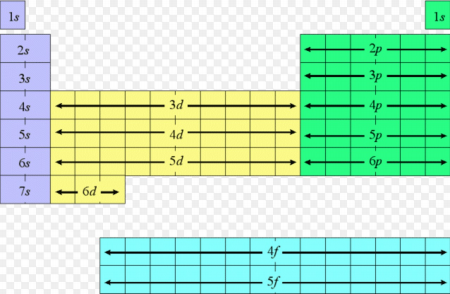∙ Hund’s Rule: single electrons occupy each orbital of the same energy before  pairing up with other electrons

Periodic Trends

∙ Core electrons: are within inner filled shells of the atoms, and they are very stable  – they don’t participate in reactions, and they can be identified by the preceding  noble gas

∙ Valence electrons: are higher in energy and are outside of the closed shells, they  determine reactivity

∙ The number of valence electrons can be determined by the element’s position on  the periodic table

∙ Trend in Atomic Radius: atomic radius increases down each group, and decreases  across each row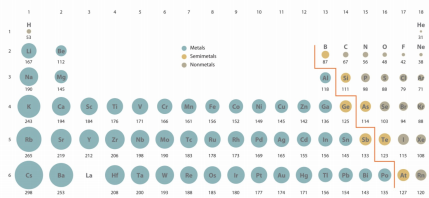∙ Atomic radius is related to the repulsion among electrons in an atom as well as the attraction between protons and electrons in an atom

∙ The atomic radius represents the state of an atom where the attraction of protons  and electrons is equal to the repulsion of electrons in the atom

∙ Atomic radius increases down each group because the total number of core  electrons increases, causing the repulsion to be stronger

∙ Going across each row, the number of protons increases while the number of core  electrons stays the same, so there is a higher net force of attraction on the valence  electrons, causing the atomic nucleus to be smaller

∙ This is known as effective nuclear charge: which depends on how much charge  from the nucleus is screened by the core electrons

∙ Zeff = number of protons in the nucleus – number of core electrons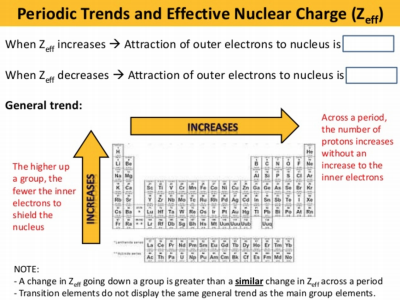∙ This represents the net charge on valence electrons from the nucleus which effects atomic radius across a row

∙ This is NOT the reason for atomic radius to increase down a group ­­­ this is  simply because there are many more electrons in each atom that are creating  repulsive forces to make the atomic radius larger

∙ Ions: are atoms in which electrons have been added or removed (altering their  charge)

∙ Cations: are formed by removing electrons (yielding a positive charge)  ∙ Anions: are formed by adding electrons (yielding a negative charge) ∙ In Li+, the entire 2s orbital is gone; also since one of the 3 electrons is lost in Li+,

there is less electron­electron repulsion ­­­ which decreases the size of the atomic  radius

∙ In anions like F­, an electron is added to the lowest energy orbital that is NOT  fully occupied ­­­ this creates more electron­electron repulsion which causes the  anion to have a larger atomic radius

∙ Ions that have the same electron configuration but are different elements are  isoelectronic (ex: Na+ and F­)

∙ Isoelectronic series: a group of ions/atoms that have the same electron  configuration but different numbers of protons

∙ For an isoelectronic series, attraction between the electrons and protons as the  charge on the nucleus increases (atomic number) ­­­ more protons in the nucleus  for the same number of electrons causes the electrons to be pulled closer to the  nucleus ­­­ leading to a smaller atomic radius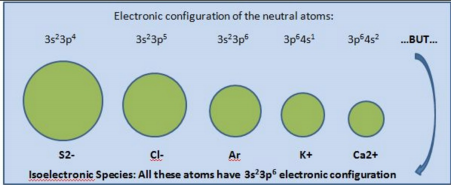∙ Ionization energy: energy required to remove an electron from an atom in the gas  phase

∙ Trend in ionization energy: ionization energy decreases down each group ∙ The effective nuclear charge is the same for Li and Na, but the outermost  electrons in Na are further from the nucleus, meaning they have less attractive  force acting on them according to Coulomb’s Law ­­­ making it easier for them to  be removed (lower ionization energy

∙ Ionization energy increases across a row ­­­ smaller atomic radius makes it more  difficult to remove electrons (so higher IE)

∙ Atomic radius and ionization energy are inversely related ­­­ this is caused by the  phenomenon of effective nuclear charge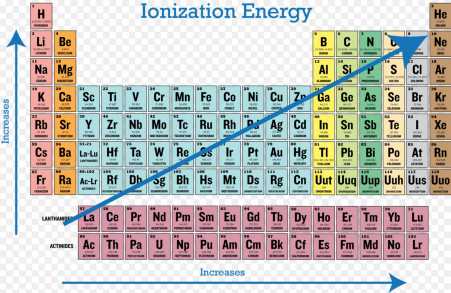∙ Ionization energy does not have a smooth rise across each row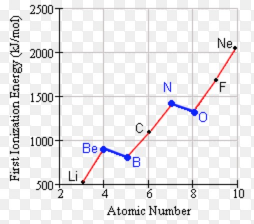∙ Boron is easier than expected to remove because it is the first electron in the 2p  orbital ­­­ it is less tightly held to the nucleus than the two electrons in the 2s  orbital

∙ In the 2p orbital this is the electron configuration of oxygen:∙ Electrons sharing an orbital have a slightly stronger repulsive force than singular  electrons that occupy orbitals, so they are slightly easier to remove (and have  slightly lower than expected ionization energy)

∙ First, second, third, etc. ionization energies refer to removing multiple electrons ∙ Higher order ionization energies increase because electrons become more and  more difficult to remove

∙ There is a large jump in ionization energy when electrons from the core are  removed (rather than valence electrons)

∙ Ionization energies can be used to determine the element by looking for the large  jump, indicating the removal of electrons from the core

∙ Ionization energies are affected by 3 factors: size of the atom (smaller size ­­­  higher IE), amount of positive charge (more positive charge, higher IE), the shell  that the electron is removed from (core electrons have higher IE than valence  electrons)

∙ The periodic change in ionization energy is evidence for atomic structure (atomic  orbitals, electron shells)

CHAPTER 3

Origins of atoms

∙ Atoms are extremely old, and they originate from stars

∙ The Big Bang happened 13.8 billion years ago

∙ Singularity: the point that big bang started from

∙ 1 picosecond later, leptons were present; 1 microsecond later, protons/neutrons  were present; few minutes later, H+, D+, He2+, Li3+ were formed via fusion  reactions

∙ These fusion reactions were able to occur because the temperature was very high  at the time

∙ Temperatures dropped and fusion reactions did not continue for 400,000 years ­­­  known as the Dark Ages

∙ Supernovas are formed by larger starts

∙ 400 million years after the Big Bang, the first stars formed

∙ The universe is expanding away from us at exponential rates

∙ Doppler effect: explains that stars with relative motion to the Earth may show  different colors due to differences in wavelength (the Doppler Effect states that  when an object emitting waves [sound, light, etc.] moves towards or away from an observer, the observer perceives shorter or longer wavelengths

∙ When the Doppler Effect occurs for stars, absorption lines in the absorption  spectrum are shifted ­­­ this is evidence for singularity if we go backwards ∙ Dark energy causes everything to move away with increasing speed ∙ CMBR (cosmic microwave background radiation): a faint glow of radiation that  permeated from the early universe which consisted of a plasma of H nuclei and  electromagnetic radiation

∙ Star formation was caused by gravitational forces after which temperature  differences throughout the universe caused matter to clump together

Nuclear Reactions

∙    Chemical reactions involve rearrangements of valence electrons, so the element never  changes; nuclear reactions involve the nucleus, which can change the element

∙    Isotopes: are atoms with the same number of protons (same element) but different  numbers of neutrons

∙    Example: 12N has 7 protons and 5 neutrons, which add up to 12

∙    Nuclear fusion: involves adding two nuclei together

∙    Nuclear fusion occurs at very high temperatures

∙    At high temperatures, electrons are stripped away from the attractive force of the  nucleus, forming plasma (fusion often occurs in stars)

∙    If there is enough kinetic energy in a two atom system, the two nuclei can move so  close to one another that the strong nuclear force comes into play and causes the  atoms to attract and fuse into one atom (this can often be achieved by adding heat) ∙    The strong force is the strongest of the 4 fundamental forces

∙    Nucleon: a proton or neutron ­­­ the strong nuclear force can only come into play at  distances of about one nucleon

∙    Once the strong nuclear force comes into effect, the potential energy of the system  decreases rapidly

∙    Potential energy curve for the two atom system during nuclear fusion:

∙    The strong nuclear force is what holds nucleons in the nucleus

∙    Nuclear fusion reactions sometimes release positrons, which are antimatter with the  same mass as an electron but a positive charge

∙    Mass­energy equivalence: E=mc2 (Albert Einstein)

∙    E is the binding energy ­­­ which is the energy released when mass is converted into  energy

∙    The energy released from nuclear reactions is the energy equivalence of the mass that  is lost (mass defect)

∙    The mass defect: mass of all the individual nucleons is less than the mass of the  nucleus

∙ Example: Each deuterium atom is formed by 1 proton, 1 neutron, and 1 electron. How much energy is released by the formation of 1 mole of deuterium?

o Given Information:

o E=mc2

o Mass defect: 0.00238 amu

o 1 amu = 1.6606 x 10­27 kg

o c = 3 x 108 m/s

o 1 mol D = 6.022 x 1023 D atoms

o SOLUTION:

o Convert mass defect to kilograms: (0.00238) x (1.6606 x 10­27 kg) = 3.95 x 10­ 30 kg

o Convert mass defect for one atom to one mole: (3.95 x 10­30) x (6.022 x 1023)  o = 2.38 x 10­6 kg

o E = mc2 = (2.38 x 10­6 kg) x (3 x 108 m/s)2 = 2.14 x 1011 J/mol

∙ Fusion reactors don’t work as sources of energy because there is a higher energy input than there is an output

∙ Nuclear fission: the process of breaking apart heavier nuclei to form lighter and more  stable nuclei + energy

∙ During the fission of 235U, the neutrons that are released start 3 more fission reactions, and those neutrons start 9 more, etc. ­­­ it becomes a chain reaction

∙ Critical mass: is the mass required for capturing the released neutron such that the  chain reaction becomes self­sustaining

∙ In nuclear reactors, people use graphite rods to absorb neutrons so the reaction stays  under control

∙ Nuclear weapons are designed for the chain reaction to lose control and explode ∙ Fission and fusion both release energy because they are forming more stable species  from less stable species

∙ Radioactivity: when the nucleus emits or captures particles or energy in the form of  electromagnetic radiation

∙ 5 common types of radioactivity: alpha particle emission, beta particles/electron  emission, positron emission, electron capture, gamma electromagnetic radiation ∙ Important to know: how nuclear reactions are different from chemical reactions,  difference between the 3 types of nuclear reactions, details on fusion covered in class

Bonding

∙ Isolated atoms do not have emergent properties: properties such as states of matter,  melting points, and boiling points – that groups of atoms have

∙ Atoms only have emergent properties when they form larger molecules or structures

∙ Nanoparticles: have different properties than bulk materials; they depend on surface  area to volume ratio; Ex: gold nanoparticles interact differently with light depending  on the size of the particle

∙ Covalent bonds form between atoms because valence electrons are attracted to the  nuclei of other atoms

∙ The interaction between two hydrogen atoms actually forms a stable bond while  helium does not, and the interaction is much stronger for hydrogen

∙ LDFs and covalent bonds are both caused by electrostatic forces, but covalent bonds  actually share electrons and are much stronger

∙ In covalent bonds, the valence electrons of one atom become attracted to the nucleus  of another, so the two atoms “fight” over the electron(s)

∙ Bond length is the most stable distance between the two atoms during the interaction  (lowest potential energy)

∙ Energy is released to the surroundings when covalent bonds form

Molecular Orbital Theory

∙ Atomic orbitals can combine constructively to form orbitals of higher energy ∙ The number of molecular orbits produced is the same as the number of atomic orbitals combined

∙ Bonding orbitals are of lower energy than the corresponding atomic orbitals ­­­  stabilizing process

∙ Antibonding orbitals are of higher energy and are destabilizing ­­­ they make the  species less stable

∙ Both bonding and antibonding orbitals are in the same place but at different energies

∙ Two hydrogen atoms form a covalent bond because their electrons fill only the  bonding orbital (stable); two helium atoms do not form covalent bonds because they

have 2 electrons in the bonding orbital as well as 2 electrons in the antibonding orbital ­­­ which cancels out the stability of the species

∙ To break a covalent bond between hydrogen atoms, enough energy must be added to  the system to raise an electron from the bonding orbital to the antibonding orbital ∙ Ex: If two oxygen atoms interact, how many molecular orbitals would be formed?  Answer: Since each oxygen atom has 5 atomic orbitals, O2 would have 10 molecular  orbitals (because there is 1 molecular orbital for every atomic orbital before the  interaction)

∙ Ex: How many bonds form between two oxygen atoms? Answer: 2 ∙ Number of bonds that form = (number of electrons in bonding orbitals – number of  electrons in antibonding orbitals)/2

∙ Atomic orbitals combine with each other to form molecular orbitals; as the number of  MOs increases, the distance between their energies decreases

∙ Bands of MOs are eventually formed (of almost continuous energy) ­­­ so a small  amount of energy is needed between MOs

∙ Valence electrons are shared by all atoms of the metal

∙ Metals are shiny because photons are absorbed and immediately fall back down to  lower energy levels (and are emitted)

∙ Metals interact with light of many wavelengths, so the metal appears shiny and  colorless

∙ Metals are shiny because photons of many wavelengths are absorbed and then emitted

∙ Band theory: valence and conduction bands overlap in metals, allowing electrons to  flow easily; in semiconductors, there is a small separation – so some energy is  required for electrons to jump energy levels

Phase Changes and Bonding

∙ Strength of attractive interactions between particles can be estimated from melting  and boiling points

∙ Continuous materials have much higher melting/boiling points than discrete materials  ­­­ covalent bonds must be overcome for carbon

∙ Covalent bonds are present only when atomic orbitals combine constructively, LDFs are present between all molecular species

∙ Carbon allotropes: different arrangements of carbon atoms

∙ Diamond is hard, translucent, and does not conduct electricity; graphite is softer and  does conduct electricity

∙ Bonding in diamonds consists of tetrahedral structures because each carbon forms 4  identical bonds to other carbons

∙ Valence Bond Theory is used to explain bonding in carbon: VB theory assumes that  atomic orbitals overlap to form bonds

∙ MO theory assumes that electrons are delocalized, but VB theory assumes that  electrons are localized in the bond

Carbon and Graphite

∙ Orbitals often hybridize (ex: in diamond or graphite) ­­­ when carbons combine, the s  and p orbitals hybridize into sp2 (graphite) or sp3 orbitals

∙ # of atomic orbitals in = # of hybrid orbitals out

∙ When carbons combine in tetrahedral geometry, sigma bonds are formed between two sp3 orbitals (in diamond)

∙ When carbons combine in trigonal planar geometry, sigma bonds and pi bonds (between the unchanged p­orbitals) are formed (in graphite)

∙ In diamond, each carbon bonds to 4 other carbons; in graphite each carbon bonds to 3  other carbons

∙ In diamond, a giant continuous tetrahedral structure is formed; in graphite, separate  sheets of trigonal planar structured carbons exist

CEM 141 EXAM 2 Study Guide

KEY CONCEPTS (Derived and adapted from the mock exam)

*All images are from internet sources (images.google.com)

• On energy diagrams, absorption is represented by an increase in energy while  emission is represented by a decrease in energy; the length of the arrow on the  diagram corresponds to the amount of energy

• One individual molecule of a substance does not have boiling/melting points because  boiling/melting points are emergent properties of groups of molecules • The major elements found throughout the human body were formed AFTER the Big  Bang – during fusion reactions in stars and stellar explosions

• Covalent bonds occur within molecules (not between molecules – that is LDFs) • LDFs can occur either between molecules, or within large molecules • Diffraction of X­rays is used to determine the structure of different types of crystals –

this is possible because EM radiation waves arrive in phase and out of phase ­­­ this  can be detected

• Diffraction (through a double slit apparatus) is also evidence for the wave nature of  light

• When electrons go from higher energy to lower energy levels, photons equal in  energy to the difference in energy levels are emitted

• Each element has specific values for its quantized energy levels, so it only emits  photons of corresponding wavelengths – so every element emits photons of different  wavelength

• Since the universe is expanding, stars are moving away from the Earth very quickly.  The Doppler Effect causes their light wavelengths to be perceived as longer than they actually are, which explains why emission and absorption spectra are shifted when  observing distant stars (red shift)

• Absorption and emission spectra are complimentary – so which ever wavelengths an  element does not absorb, it emits

• Valence electrons can be determined from the periodic table based on how far away  an element is from the last noble gas – for example, Chlorine has 7 valence electrons  because it is 7 elements after Neon (the last noble gas), meaning that the rest of its  electrons are core electrons (10 core electrons)

• When electrons move from lower energy levels to higher energy levels, the atom  absorbs photons equal in energy to the difference in the two energy levels (see the  first image)

• Atomic radius is related to the strength of the attractive interactions between electrons and the nucleus. When the effective nuclear charge is higher, atomic radius is smaller  (this is why atomic radius decreases across a row)

• Know the potential energy curve for fusion reactions

• Fusion reactions involve grouping small atoms (like hydrogen isotopes) together,  while fission reactions involve splitting larger atoms (like uranium isotopes) apart • The terms solid, liquid, and gas only have meaning if there are multiple particles • E=hc/λ is an equation that can be adapted from E=hυ and c=λυ to relate energy and  wavelength

• Hydrogen atoms are not separated at temperatures below about 6000K because the  covalent bonds hold hydrogen molecules together so tightly

• Wavelengths of electrons are similar to the size of atoms

• In the photoelectric effect, threshold frequency is what allows some frequency of  light to emit photons from a surface of a metal but not others. This phenomenon of  threshold frequency is NOT related in any way to intensity

• When covalently bonded molecules such as hydrogen boil, the covalent bonds are  NOT broken – only the LDFs

• Large jumps in successive ionization energies indicate that core electrons are being  removed (which are held to the nucleus much more strongly) – this pattern in  ionization energy can be used to determine the identity of elements

• According to Coulomb’s Law, the core electrons are much more tightly bound to the  nucleus than the valence electrons – this explains the jump in ionization energy  • Ionization energies are also related to the quantized energy levels for electrons,  because ionization energies are discrete, just like quantized energy levels • Nuclear fusion is inefficient because it requires a lot of energy for to overcome the  electrostatic repulsion to fuse atoms together (more energy input than output – net  loss)

∙ Continuous materials such as carbon have much higher melting points than discrete  materials such as molecular hydrogen because carbon has covalent bonds that must be broken in order for it to melt or boil, whereas molecular hydrogen only requires the  breaking of LDFs

∙ Metals appear to be shiny because their near­continuous energy bands allow them to  absorb photons and re­emit them directly after

∙ In an isoelectronic series, atoms with the most protons are the smallest because they  have the greatest effective nuclear charge (since their electron configurations are  identical)

∙ Diamonds do not conduct electricity because all of their electrons are localized (so  there is a lack of free electrons)

∙ Diamonds have high melting points because all of the covalent bonds between  carbons form a giant structure, making it extremely difficult to break all of the  covalent bonds

∙ Metals have delocalized electrons (allowing them to conduct electricity better) while  structures such as diamond have localized electrons

∙ Down each group, atomic radius increases due to more electron­electron repulsion ∙ Across each row, atomic radius decreases due to higher effective nuclear charge (more protons in the nucleus attracting the same number of core electrons)

∙ λ=h/mv can be used to determine the wavelength of any object with mass and  velocity (de Broglie’s wavelength)

∙ When light is treated as a particle, increased intensity means more photons. When  light is treated as a wave, increased intensity means greater amplitude of the wave ∙ Diamond has a large tetrahedral structure of carbons whereas graphite has  disconnected segments of carbons

∙ A substance can boil while be heated up due to molecules gaining kinetic energy from colliding with the edges of the container (thermal energy transferred to kinetic  energy) ­­­ they can then overcome electrostatic forces of attraction and escape into  the gas phase

∙ Ionization energy is higher for atoms with smaller nuclei (IE and atomic radius are  inversely related)

∙ Wavelength and frequency are inversely related (c=λυ)

∙ When covalent bonds form, stability increases – and it is a result of electrons of  atoms being attracted to the nuclei of other atoms

∙ Molecules held together by covalent bonds will always have higher melting points  than materials held together by LDFs

∙ s orbitals are spherical, p orbitals are two areas of electron density separated by a  node, d orbitals have four regions of electron density

∙ Electron configurations can be shortened by referring to the last noble gas in  parenthesis

∙ Hybridized orbitals: sp3 forms tetrahedral structure; sp2 forms trigonal planar structure

Page ExpiredIt looks like your free minutes have expired! Lucky for you we have all the content you need, just sign up here